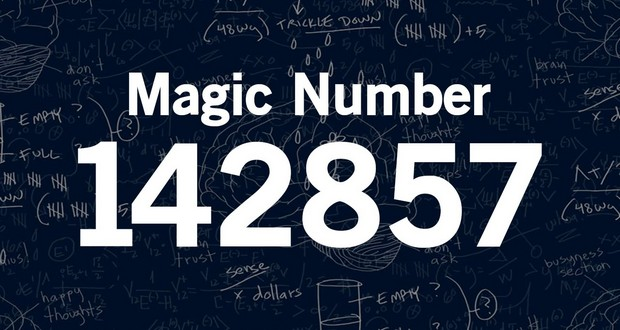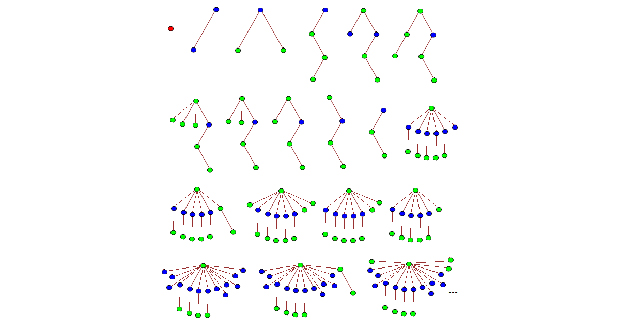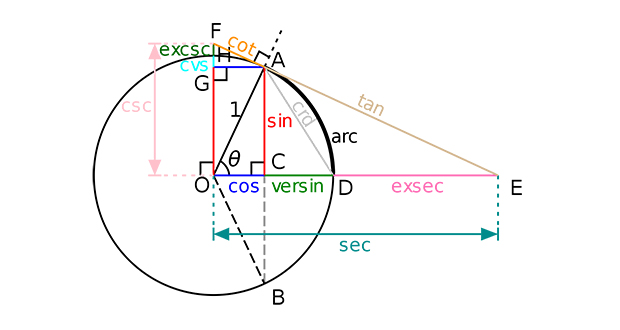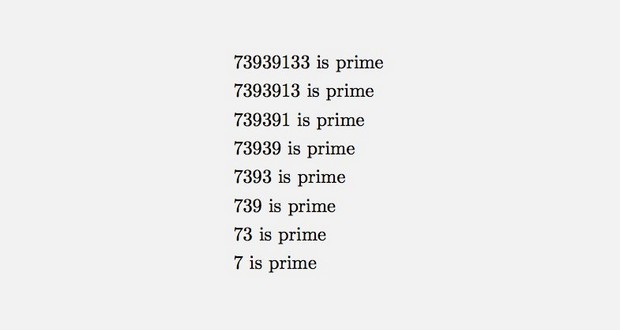# 30 Facts About Numbers That’ll Make You Feel Like a Mathematical Genius

## 11142,857The number 142,857, when multiplied by 1,2,3,4,5, or 6, is an anagram of itself. The fractions 1/7 through 6/7 also are forms of 142857, and the right six digits of any higher multiple plus the remaining left digits will equal an anagram of 142857.

## 12Fibonacci sequenceThe inverse of 999,999,999,999,999,999,999,998,999,999,999,999,999,999,999,999 gives the fibonacci sequence in 24 digit strings.

## 13Percent symbolThe percent symbol (%) is supposed to be a fraction of zero over zero. There are higher symbols such as the permille sign (‰) and others for smaller percentages.

## 14Tree(3)Tree(3) is a number so large that Graham's number of people wouldn't be able to begin calculating it. The only thing we know about Tree(3) is that it is finite. We do not know what it is or even how many digits there are.

## 15Lucky numberThe number 8 is such a lucky number in China that the Chinese government scheduled the Beijing Olympics to begin 8 seconds after 8:08 on 8/8/2008.

## 16TrigonometryTrigonometry was invented by Muslim mathematicians as a way for worshippers to calculate the direction to Mecca for prayer.

## 17Kaprekar's constant6174 is Kaprekar's constant. If you have a 4 digit number with at least 2 distinct digits, order the numbers within it in ascending and descending order, subtract the smaller number from the larger, and repeat with the result, you'll eventually always end up with 6174.

## 187393913373939133 is the largest known prime number that, if you keep removing digits from the right, will always leave a prime number.

## 19Abū al-Wafā' BūzjānīAbū al-Wafā' Būzjānī was a Persian mathematician and astronomer who worked in Baghdad and made important innovations in spherical trigonometry.

## 20Henri PoincaréIn 1887, a mathematician named Henri Poincaré received a prize for his work on the stability of the solar system. A year later, after finding an error in his own work, he published a paper contradicting it, which led to the development of chaos theory.